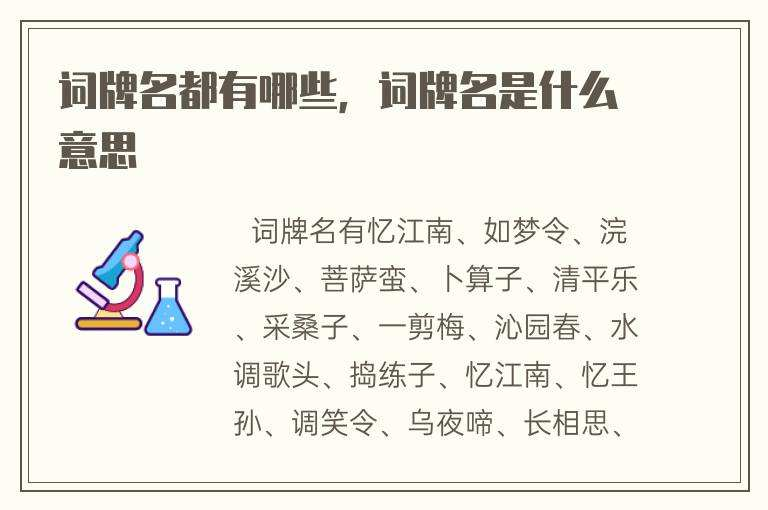# 有哪些词牌名(有哪些词牌名的诗?)

(平)平(仄)仄仄平平，(仄)仄平平(仄)仄平。(仄)仄平平(仄)仄平。仄平平。(仄)仄平平(仄)仄平。(仄)仄(仄)平平仄，(仄)仄(仄)平平仄。(仄)仄仄平平，(仄)仄(仄)平平仄。平仄，平仄（叠句），(仄)仄仄平平仄。

[平]平[平]仄平平，仄平平。[仄]仄[平]平平仄、仄平平。[仄][平]仄，[仄][平]仄，仄平平。[仄]仄[平]平平仄、仄平平。

(平)平(仄)仄平，(仄)仄平平仄。(仄)仄仄平平，(仄)仄平平仄。(平)平(仄)仄平，(仄)仄平平仄。(仄)仄仄平平，(仄)仄平平仄。

(仄)仄平平，(仄)平(平)仄平平仄。(仄)平(平)仄，(平)仄平平仄。(仄)仄平平，(仄)仄平平仄。平平仄，(仄)平(平)仄，(平)仄平平仄。

(仄)仄平平仄仄平，(平)平(仄)仄仄平平。(平)平(仄)仄仄平平。(仄)仄(平)平平仄仄，(平)平(仄)仄仄平平。(平)平(仄)仄仄平平。

(平)平(平)仄平平仄，(平)平(仄)仄平平仄。(平)仄仄平平，(仄)平平仄平。(仄)平平仄仄，(仄)仄平平仄。(平)仄仄平平，(仄)平平仄平。

(仄)仄仄平平，(仄)仄平平仄。(仄)仄平平仄仄平，(仄)仄平平仄。(仄)仄仄平平，(仄)仄平平仄。(仄)仄平平仄仄平，(仄)仄平平仄。

(平)平(仄)仄平平仄，(仄)仄平平。(仄)仄平平，(仄)仄平平(仄)仄平。(平)平(仄)仄平平仄，(仄)仄平平。(仄)仄平平，(仄)仄平平(仄)仄平。

(平)平(仄)仄，(仄)仄(平)平平仄仄。(仄)仄平平，(仄)仄平平(仄)仄平。*(平)平(仄)仄，(仄)仄(平)平平仄仄。(仄)仄平平，(仄)仄平平(仄)仄平。

1.(平)平(仄)仄仄平平。(仄)仄仄平平。(平)平仄平仄，(仄)仄仄、仄平平。平仄仄，仄平平，仄平平。仄平平仄，(仄)仄平平，仄仄平平。

2.(平)平(仄)仄仄平平。(仄)仄仄平平。(平)平仄仄平仄，(仄)仄仄平平。平仄仄，仄平平，仄平平。仄平平仄，(仄)仄平平，仄仄平平。

(平)平(仄)仄，(仄)仄平平仄。(仄)仄(平)平平仄仄，(仄)仄(平)平(仄)仄。(平)平(仄)仄平平，(平)平(仄)仄平平。(仄)仄(平)平(仄)仄，(平)平(仄)仄平平。

(仄)仄仄平平，(仄)仄平平。(平)平(仄)仄仄平平。(仄)仄(平)平平仄仄，(仄)仄平平。

(仄)仄仄平平，(仄)仄平平。(平)平(仄)仄仄平平。(仄)仄(平)平平仄仄，(仄)仄平平。

(仄)仄平平(仄)仄平，(平)平(仄)仄仄平平。(平)平(仄)仄平平仄，(仄)仄平平(仄)仄平。

(平)平(仄)仄平平仄，(仄)仄平平仄。

(平)平(仄)仄仄平平，(仄)仄(平)平(仄)仄仄平平。

(平)平(仄)仄平平仄，(仄)仄平平仄。

(平)平(仄)仄仄平平，(仄)仄(平)平(仄)仄仄平平。

(仄)仄仄平平，(仄)仄平平仄仄平。(仄)仄(平)平平仄仄，平平。(仄)仄平平仄仄平。(仄)仄仄平平，(仄)仄平平仄仄平。(仄)仄(平)平平仄仄，平平。(仄)仄平平仄仄平。

(平)平(仄)仄平平仄，(仄)仄(平)平平仄仄。(平)平(仄)仄仄平平，(仄)仄(平)平平仄仄。(平)平(仄)仄平平仄，(仄)仄(平)平平仄仄。(平)平(仄)仄仄平平，(仄)仄(平)平平仄仄。

（仄）平（平）仄，（平）平（仄）仄平平（仄）。（平）平仄仄（平）平（仄），仄仄平平、仄仄平平仄。（仄）仄（平）平平仄（仄），（平）平仄仄（平）平（仄）。平平（仄）仄（平）平仄，仄仄平平、仄仄平平仄。

(仄)仄平平，(平)平(仄)仄，(平)平(仄)仄平平仄。(平)平(仄)仄仄平平，(平)平(仄)仄平平仄。

(仄)仄平平，(平)平(仄)仄，(平)平(仄)仄平平仄。(平)平(仄)仄仄平平，(平)平(仄)仄平平仄。[仄]仄平平仄仄平，[仄]仄平平，[仄]仄平平，[平]平[仄]仄仄平平。[仄]仄平平，[仄]仄平平。[仄]仄平平仄平平。[仄]仄平平，[仄]仄平平，[平]平[平]仄仄平平。[仄]仄平平，[仄]仄平平。

(仄)仄(平)平平仄仄，(平)平(仄)仄平平。(平)平(仄)仄仄平平。(平)平平仄仄，(仄)仄仄平平。(仄)仄(平)平平仄仄，(平)平(仄)仄平平。(平)平(仄)仄仄平平。(平)平平仄仄，(仄)仄仄平平。

(仄)仄(平)平平仄仄，(平)平(仄)仄平平仄。(仄)仄(平)平平仄仄。平(仄)仄，(平)平(仄)仄平平仄。仄)仄(平)平平仄仄，(平)平(仄)仄平平仄。(仄)仄(平)平平仄仄。平(仄)仄，(平)平(仄)仄平平仄。

[仄]仄[平]平仄仄平，[平]平[仄]仄仄平平。[仄]仄[平]平平仄仄，平仄，[平]平[平]仄仄平平。[仄]仄[平]平平仄仄，平仄，[平]平[仄]仄仄平平。[仄]仄[平]平平仄仄，平仄，[平]平[仄]仄仄平平。

(仄)仄平平，(仄)仄(仄)平平仄。仄平平、平平仄仄。平平平仄，仄平平仄(上三下二)。仄平平、仄平平仄。平平(仄)仄，仄仄(仄)平平仄。仄平平、平平仄仄。平平平仄，仄平平平仄（上三下二）。仄平平、仄平平仄。

(平)平(仄)仄平平仄，仄(仄)仄平平仄（ 上三下三）。(仄)仄(平)平平仄仄。(仄)平平仄，(仄)平平仄，(仄)仄平平仄。(平)平(仄)仄平平仄，(仄)仄(平)平仄平仄。(仄)仄(平)平平仄仄。(仄)平平仄，(仄)平平仄，(仄)仄平平仄。

《水调》数声持酒听，午醉醒来愁未醒。送春春去几时回？临晚镜，伤流景，往事后期空记省。沙上并禽池上瞑，云破月来花弄影。重重帘幕密遮灯，风不定，人初静，明日落红应满径。

(平)平(仄)仄仄平平。仄平平，仄平平，(仄)仄平平，仄仄仄平平。(仄)仄(平)平平仄仄，平仄仄，仄平平。(平)平(仄)仄仄平平。仄平平，仄平平。(仄)仄平平，仄仄仄平平。(仄)仄(平)平平仄仄，平仄仄，仄平平。

(仄)仄平平，(平)(平)仄、(平)平(仄)仄。(平)(仄)仄、(仄)平(平)仄，(仄)平(平)仄。(仄)仄(平)平平仄仄，(平)平(仄)仄平平仄。仄(仄)平、(仄)仄仄平平，平平仄。(仄)(平)仄，平(仄)仄；(平)(仄)仄，平平仄。仄平平仄仄、仄平平仄。(仄)仄(平)平平仄仄，(平)平(仄)仄平平仄。仄(平)平、(仄)仄仄平平，平平仄。

1.投稿邮箱：844418467@qq.com.

2.入选要求：精选绝句20首，律诗或词10-15首，文章类一至数篇。

3.关于打赏：群内诗友集作品归平台，个人专辑全部归作者安排。